# THE TEMPORAL MULTIDIMENSIONAL MODEL

In the multidimensional model developed by Hurtado and the authors (Hurtado, Mendelzon, & Vaisman, 1999), dimensions are defined as non-temporal structures like the geography dimension of Figure 1. A dimension in this model has a schema, and instances associated to this schema which may evolve through time, although dimension versioning is not supported. In the Temporal Multidimensional Model we present in this section, we timestamp dimension elements at the schema and instance level in order to keep track of the updates that occur during the dimension's lifespan.

We will consider time as discrete; that is, a point in the timeline, called a time point, will correspond to an integer. We will also assume, except when noted, instant t0 to be the dimension's creation instant.

Temporal Dimensions. The following sets are defined: a set of level names L, where each level l L is associated with a set of values dom(l); a set of attribute names A, such that each attribute a A is associated with a set of values dom(a); a set of temporal dimension names TD; and a set of fact table names F.

Definition 1 (Temporal Dimension Schema): A temporal dimension schema is a tuple (dname, L, λ, , A, >>, μ) where:

• dname TD is the name of the temporal dimension.

• μ is a level in the Time dimension. Intuitively, μ defines the granularity of the dimension dname. It represents transaction time.

• L L is a finite set of levels, which contains a distinguished level name All, s.t. dom(All) = {all}. This distinguished level is considered valid during the complete lifespan of the dimension.

• "λ" is a function with signature dom(μ) L, defining the instants when each level was part of the dimension.

• "" is a function with signature dom(μ) 2LxL, such that for each t dom(μ), , is a relation such that *t, the transitive and reflexive closure of t, is a partial order, with a unique bottom level, 1inf λ (t), and a unique top level, All, where, for every level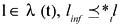and *t All hold.

• A is a finite set of attributes.

• "" is a function with signature dom(μ) x A L.For every level l λ (t), a t l means that if the function is applied to an attribute a, it returns the level l such that attribute a belongs(ed) to level l at time t.

The structure introduced by Definition 1 suggests that, given an appropriate language, it would be possible to query the schema of a dimension (using the functions and ). Note that linf is unique at any time instant t, although it may not be the case at different times. Also observe that taking a snapshot of the schema at a given time t, we get a non-temporal dimension schema.

Notation: In the figures of this chapter, a label ti associated to an edge in a graph, will mean that the edge is valid for all t ti, and a label t*i, that the edge was valid for all t < ti. If an edge has no label, it is considered valid for all of the dimension's lifespan. An interval [ti, tj) means that the edge is valid from t ti, to t < tj.

Definition 2 (Temporal Dimension Instance): A temporal dimension instance is a tuple (D, TRUP, TDESC), where D is a temporal dimension schema, and:

• TRUP (temporal roll-up) is a set of functions, satisfying the following conditions:

• For every instant t dom(μ), and for each pair of levels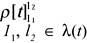such that l1 tl2t, there exists in TRUP a roll-up function: dom (l1) dom(12). Thus, a function is defined for every snapshot taken at any instant t dom(μ).

• For every instant t in the dimension's lifespan, and for every pair of paths τ1 and τ2 in the graph with nodes in λ(t) and edges in t, such that τ1 = < l1, l2, , lk, ln >, and τ2 = < l1, l'2, , l'k, ln >, we have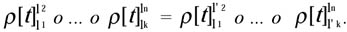• At every instant t of the dimension's lifespan, and for each triple of levels l1, l2, l3 λ(t) such that l1 t l2 and l2 t l3, ran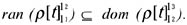• TDESC (temporal description) is a set of functions such that for every instant t dom(μ), and for each level l λ(t) and for each attribute a such that a >>t l, there exists in TDESC a function with signature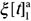: dom(l) dom(a).

We will call the second condition in Definition 2 snapshot consistency.

Example 1: Figure 3 depicts a schema and an instance for a dimension Store. Here, dname = Store, L = {storeId, storeType, city, region, All}, μ = day. Initially "" contains the following pairs: storeId city, city region, region All. The addition of a new level storeType above level storeId at time d1 will modify "" as follows: = {storeId city, city region, storeId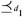storeType, region All, storeType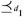All}.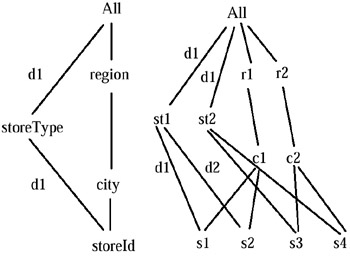Figure 3: The Temporal Dimension "Store"

Figure 3 also shows a temporal dimension instance for dimension Store. We see that the roll-up functions with no label are valid for the whole lifespan of Store, while for example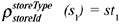is valid from d1on.

Definition 3 (Active Instance Set): Given a temporal dimension d, a level l L, and an instant t, the set of elements belonging to dom(l) at time t is called the Active Instance Set of l. We denote it Ainstset (l,t).

Example 2: In Figure 3, Ainstset (stereId, d) = {sl, s2, s3, s4}, for all d. However, deleting s1 at time d3 will yield Ainstset (storeId, d3)= {s2, s3, s4}.

The previous definitions set the basis for a temporal data warehouse. We will introduce the idea through the following example.

Example 3: Figure 4 shows a sequence of updates to a temporal dimension Product. Initially, Product consisted only of level itemId, and the distinguished level All. After that, the brand was added to the dimension, although the initial state is not lost (Figure 4(b)). Later, the type of the item is inserted, with level name itemType. The company to which an item belongs is also added above level brand at time d5, and level brand is deleted at time d9 (Figure 4(d)). Note that an edge is added from level itemId to level brand, valid from time d9on.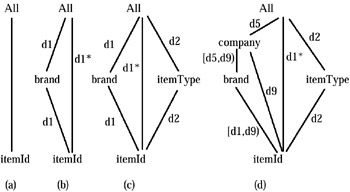Figure 4: A Series of Updates to Dimension "Product"

A slice or snapshot (in temporal database terminology) of a dimension, taken at a given instant t, defines the state of the dimension at that time. For instance, Figure 5 shows a snapshot of dimension Product at d4.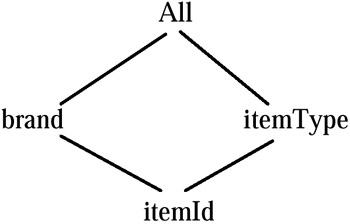Figure 5: A Snapshot at "d4"

Definition 4 (Temporal Fact Table): A temporal fact table schema is a tuple s = (fname, f, m, μ), where m is a level name, called the measure of the fact table μ, is a level in the Time dimension, and f is a function with signature dom(μ) 2L.

Given a temporal fact table schema (fname, f, m, μ), a set of levels L in the range of f, and a level μ in the Time dimension, a mapping from each level li (L μ) to dom(li) is called a point.

Given a temporal fact table schema s = (fname, f, m, μ), a temporal fact table instance over it is a partial function named f name which maps points of s to elements in dom(m).

Definition 5 (Temporal Base Fact Table): Given a set D of temporal dimensions, a temporal base fact table is a temporal fact table with schema (fname, fD, m, μ), where f is a function with signature dom(μ) 2L, such that for each t dom(μ), every level in fD(t) is a bottom level of the dimension it belongs to. Thus, a temporal base fact table is a temporal fact table such that its attributes are the bottom levels of each one of the dimensions in D.

Example 4: Given D = {Store, Product} where dimensions Store and Product are those of Figures 3 and 4 respectively, the Temporal Base Fact Table associated to D would have fD(t) = {storeId, itemId}for all t. If updates occur such that, for instance, at time d12, brand becomes the bottom level of dimension Product, fD(d12) = {storeId, brand}.

Definition 6 (Temporal Multidimensional Database):

• A temporal multidimensional database schema, denoted Bs, is a pair (Ds, Fs), where Ds is a set of temporal dimension schemas, and Fs is a set of temporal fact table schemas.

• A multidimensional database instance I(B), is a tuple (FI, DI), where DI and FI are dimension and fact table instances defined as above.

#### Valid and Transaction Times in the Temporal Multidimensional Model

In temporal databases, in general, instances are represented using valid time, this is, the time when the fact being recorded became valid. On the contrary, a database schema holds within a time interval that can be different from the one within which this schema was valid in reality. Changes in the real world are not reflected in the database until the database schema is updated. Schema versioning, thus, is often related with transaction time. In our temporal multi-dimensional model, things are different than above: an update to a dimension schema modifies the structure as well as the instance of the dimension. Figure 3 shows a dimension where schema and instance updates occurred. When storeId becomes generalized to storeType, the associated roll-ups must correspond to the instants in which they actually hold. Thus, we consider that temporal dimensions are represented by valid time. It is straightforward to extend the model for supporting temporal dimensions with valid and transaction times.

Fact table instances can be represented in our model using valid and transaction times. In order to accomplish this, two time dimensions must be defined. Fact table schema versioning is represented using transaction time. When a bottom level of a dimension is deleted or a level is added below it, the schemas of the associated fact tables change and new versions are defined for them.

### TOLAP: A Temporal OLAP Query Language

In the previous section we introduced a model accounting for temporal dimensions, i.e., dimensions that evolve across time, allowing us to keep track of the dimension's history. Let us call this dimension versioning. We will show that typical OLAP queries involving temporal dimensions admit different interpretations, which should be considered in order to give the user the correct answers to queries. Further, we will introduce the TOLAP query language, which supports the model presented in the previous section.

#### Do We Need a Temporal OLAP Query Language?

One might argue, at first sight, that a generic temporal query language like TSQL2 (Snodgrass, 1995) could be used instead of defining a special-purpose one like TOLAP. There are two main reasons supporting the idea of introducing a new language. First, a language designed specifically for the multidimensional model makes typical OLAP queries much more concise and elegant. In a generic language, queries would have to be laboriously encoded using detailed knowledge of the low-level relational structures used to encode the dimensional data. Second, the best-known temporal query languages such as TSQL2, support only a minimal level of schema versioning (Snodgrass, 1995, p.29).

Another alternative would have been adding temporal features to other languages with schema management features, such as HiLog (Chen, Kifer, & Warren, 1989) or SchemaLog (Lakshmanan, Sadri, & Subramanian, 1993). Again, using a language specifically designed for OLAP yields much simpler syntax and semantics, and just the high-order features that are needed to support schema evolution.

The following example will show that a temporal OLAP query language is needed in order to capture the particular characteristics of OLAP queries expressed over a set of temporal dimensions.

Let us consider again a retail data warehouse, with a set of temporal dimensions D = {Product, Store}, and a base fact table with schema (Sales, f, sales, day). Dimensions Store and Product are the ones in Figures 3 and 6, respectively. The dimension levels in the fact table are {itemId, storeId}, the bottom levels of the dimensions in D. Notice that in Product, item i1 has type ty1 until day d4, and type ty2 since then. For the Time dimension we assume: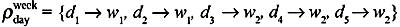(and the roll-ups from week to All). Finally, we have the following instance for the Sales fact table (day is displayed for the sake of clarity, but could have been omitted, like in TSQL2):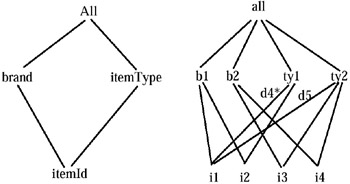Figure 6: Dimension Product for the Running Example

Let us now suppose the query: "List the weekly total sum of sales, by city and item type." Two interpretations could be given to this query. The usual one would compute the sum of sales considering the type an item had when it was sold. In this case, for instance, item i1 would contribute to the aggregation in the following way: the first three tuples, with a total of 800, will add to the group {ty1, c1, w1}, while the last one will contribute to {ty2, c1, w2}.

itemId

storeId

day

sales

i1

s1

d1

600

i2

s2

d1

100

i2

s1

d2

100

i3

s2

d2

100

i3

s3

d3

100

i3

s4

d4

100

i1

s1

d5

100

The second interpretation, the only one currently supported by non-temporal systems, would compute the sum of the sales considering that each sold item has the current type, regardless of the time the sale occurred. The result a user would get under this interpretation is given by the table below, and was computed in the following way: the roll-up function for every occurrence of item i1 is set to:

The results will be given by the following table.

itemType

city

week

sales

ty1

c1

w1

800

ty2

c1

w1

100

ty2

c2

w2

200

ty2

c2

w2

100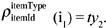Thus, all the i1 tuples will contribute to type ty2. For instance, the first tuple will now contribute to the group {ty2, c1, w1}. The following table shows the result the user would obtain under the second interpretation.

#### TOLAP by Example

We will first present TOLAP by means of examples, and then define its syntax and semantics. It is interesting to notice that the queries we will present operate at a high level of abstraction, without requiring low-level knowledge about the database design that underlies the dimensional model.

We will work with a set of dimensions D = {Store, Product}, where Store is the dimension in Figure 3 and Product is the dimension depicted in Figure 4; the base fact table Sales is the one introduced at the beginning of this section.

itemType

city

week

sales

ty1

c1

w1

200

ty2

c1

w1

700

ty2

c2

w2

200

ty2

c2

w2

100

Simple Queries. We begin with queries not involving aggregates.

Example 5: A query returning the sales for stores in Buenos Aires, on a daily basis, will be expressed in TOLAP as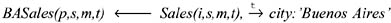In TOLAP, the query above returns the tuples in Sales such that s rolled up to ‘Buenos Aires’ at the time of the sale, where s represents an element in the instance set of the lowest level of the dimension Store. The query is interpreted as follows: a tuple in Sales will be in the result if an item i was sold in store s on a day t if s was settled in Buenos Aires on that day (recall that the granularity of the fact table is day ).

We assume a fixed order of the attributes in the base fact tables. For instance, in the base fact table Sales, the first position from the left will always correspond to dimension Product.

Queries with Aggregates. In order to address queries involving aggregation, we adapted a non-recursive data log with aggregate functions (Consens & Mendelzon, 1990) which, in turn, was based on the approach of Klug's relational calculus with aggregates (Klug, 1982).

Example 6: Consider the query: "list the total sales per item and region," where we want aggregates to be computed using temporally consistent values (i.e., a sale in a given store is credited to the region that corresponded to that store at the time of the sale).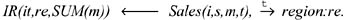This query is interpreted as follows: let us suppose a pair of tuples t1: < i1, TaosBranch, 100, 10/10/01 >, and t2:< i1, DallasBranch1, 100, 10/10/01 >, in fact table Sales; moreover, assume that the store branches identified by TaosBranch and DallasBranch1 were assigned to the southern region on October 10, 2001. As the expression s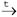region:re will evaluate to true when variables s, t, and re become instantiated with values TaosBranch (or DallasBranch1), 10/10/01, and Southern, respectively, the tuple < i1, Southern, 200 > will be in the result.

It is worth noting that in case the time granularities of the fact table Sales and the Store dimension differ (for instance, if the dimension's granularity was month), the user would not have to make the granularity conversion explicit, allowing a limited form of "schema independence." Later examples show the use of variables that range over level names, pushing this independence farther.

Example 7: We now introduce descriptive attributes of dimension levels. Suppose we want the total sales by store and brand, for stores with more than 90 employees. Assume that the level storied is described by an attribute nbrEmp (number of employees).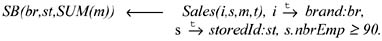Metaqueries.TOLAP also allows querying the system's metadata, supporting queries with no fact table in the body of the rules. We call these queries metaqueries.

• "Give me the time instants at which store s1 belonged to the Southern region," expressed as: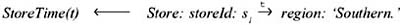* Note that we must specify the name of the dimension in the atom Store: storeId: ‘s1,’ because there is no fact table in the body of the rule to which the dimension's bottom level could be bound.

• "List the months when ‘Southern’ was not a valid region."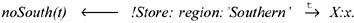In the example above, variable X ranges over level names in the Store dimension. Variable x is bound by the values in the levels of the dimension. The domain of t is the lifespan of the dimension referenced in the metaquery. For every instantiation of the variables X,x, and t, which makes the body rule of the rule true, the time instant t is listed.

• "Were products categorized by brands two years ago?"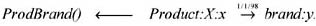Here again X ranges over level names. The expression above means that if any element, in any level in the Product dimension, is rolled-up to an element in level brand at the required date, the answer to the query will be "yes."

• "How were customers classified three years ago?"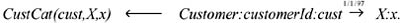#### Data Warehouse Versioning in TOLAP

Earlier, we showed that our model supports different versions of a dimension schema over time (in temporal database terminology this is called schema versioning). For instance, consider an alternative history for dimension Store in our running example, which is depicted in Figure 7. We can see that the bottom level of Store was initially city, and at time d5 storeId was inserted below it. Also assume that the fact table depicted below was in effect before d5.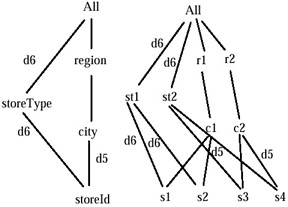Figure 7: Data Warehouse Versioning

itemId

city

sales

day

i1

c1

100

d1

i2

c2

100

d2

i1

c3

100

d3

i2

c1

100

d1

After the update, the sales in the following table occurred (notice the new structure of the fact table).

itemId

storeId

sales

day

i1

s4

100

d6

i2

s2

100

d6

i1

s3

100

d7

i2

s1

100

d7

In TOLAP, an element not defined at a given instant will not contribute to the result. For instance, given the query "list the total sum of sales by brand and storeId," we have: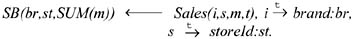The expression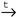storeId:st means that if an element in any level, which was once a component of a base fact table, rolled-up to level storeId at time t, it contributes to the aggregation. Thus, the sales made before d6 will not contribute to the aggregation in the head (conditionstoreId:st will not be satisfied). Analogously, a query like "total sales by store and itemId" would return exactly the instance of the second fact table above. This query is expressed: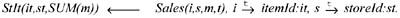For the dimension Product, Figure 4 shows that at time d5, level company was added above level brand. Given the query, "total sales by company and region," the sales occurring before d5 will not contribute to the aggregation, as company was not a level of the dimension at that time. The query reads in TOLAP: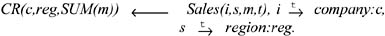Finally, at time d9, level brand was deleted from Product. The query "total sales by brand and region" is expressed in TOLAP as: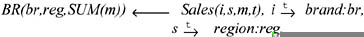Any sale that occurred after d9 will not be considered, as brand is not a level of the dimension anymore.

#### TOLAP Programs

A TOLAP program allows computing the result of a rule and using it as a predicate in the body of another rule. The predicate's name is the name of the predicate in the head of the rule which computes it.

For instance, let us suppose the following query: "List the total sales by brand, for those brands that sold more than \$100,000 in Buenos Aires." We want to answer this query precomputing a view holding the total sales in Buenos Aires, by brand.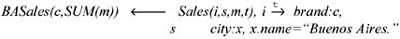Then, we compute the query, using predicate BASales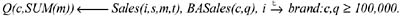For each brand in dimension Product matching a brand in BASales, in the second rule, if the amount sold was greater than 100,000, the sales contributes to the aggregation.

#### Syntax

We will formally define the syntax of a TOLAP rule. We will first give some definitions which will be used below, and then formalize the concepts previously introduced in the section, TOLAP by example.

Preliminary Definitions. Given a set T, and a discrete linear order <, with no endpoints, we define a point-based temporal domain as the structure TP = (T, <). Analogously, given TP = (T, <), we define the set I(T) = {(a, b) | a b, a, b T {−∞, +}}. Let us denote by θ the set of the usual interval comparison operators. Then, TI = (I(T), θ) is an interval-based temporal domain corresponding to TP. The roll-up functions in TOLAP will be defined over TP.

Atoms, Terms, Rules, and Programs. Assume Bs (Ds, Fs) and I (B) represent a multidimensional database schema and instance, respectively, as already defined. Let VL and VD be a set of level and data variables, respectively. We have also the sets CL and CD of level and data constants, respectively. Let P be a set of predicate names and FAg a set of aggregate function names.

Definition 7 (Terms):

• A data term is either a variable in VDor a constant in CD;

• a roll-up term is an expression of the form d:X:x, X:x, or x, where X is a level name variable in VLor constant in CL, x is a data term, and d is a constant in CL;

• a descriptive term is an expression of the form x.a where x and a are data terms;

• an aggregate term is an expression of the form f(d) such that f is a function name in FAg and d is a data term.

A term is a data, roll-up, descriptive, or aggregate term.

Definition 8 (Atoms):

• A fact atom is an expression of the form F(X1, , Xn, M, t), where F is a fact table name in Fs,and X1, , Xn, M and t are data terms;

• a roll-up atom is an expression of the form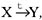, or X Y, where X and Y are roll-up terms, and t is a data term;

• a descriptive atom is an expression of the form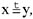where x is a descriptive term, and y and t are data terms;

• an aggregate atom is of the form Q(R,,Z) s.t. Q P, and R,,Z are data terms s.t. at least one is an aggregate term;

• a constraint atom is an expression t1 θt2, where t1 and t2 are data terms, and θ is one of {<, =};

• if g : N × × N N is a scalar function, g(n1, , nm), where ni are data terms, is a scalar atom;

• an intentional (extensional) predicate atom is an expression of the form p (X, , Z) where X,Y,Z are data terms, and p is an intentional (extensional) predicate name in P. In what follows, we will refer to these kinds of atoms simply as predicate atoms when possible.

An atom is a fact, roll-up, descriptive, aggregate, constraint, scalar, or predicate atom. An expression !t1, where t1 is an atom, is a negated atom.

Definition 9 (TOLAP Rules): A TOLAP rule is a formula of the form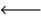A1, A2, , An, where A is an intentional (possibly aggregate) positive atom, and Ai, i = 1n are non-aggregate atoms. A TOLAP rule Γ satisfies the following conditions:

• if a variable appears in the head of the rule, it must also appear in its body;

• for every level/data variable v, all the roll-up terms in which v appears are associated to the same dimension; moreover, all the variables in a roll-up atom belong to the same dimension;

• if there is an aggregate atom Q(al, , an) in the head of the rule, for all atoms in the body, of the form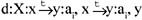is a constant data term (thus, aggregation is performed over constant level names);

• every variable x in a roll-up atom of the form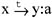must appear in a fact atom in the body of the rule;

• if x.a is in the body of Γ, at least one roll-up term in the body is of the form d:X:x, X:x, or x;

• every variable appearing in a predicate atom must appear in a fact or roll-up atom in the body of the rule;

• in a roll-up term of the form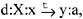, d is a constant data term;

• if there is no fact atom in the body of Γ, all the roll-up atoms must be of the form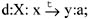• In a negated fact atom, at least one of its terms must be a constant in CD;

• if t1 θ t2 is a constraint atom, t1 and t2 are both variables in a fact, roll-up, or descriptive atom in the body, or one of them is a constant in CD and the other is a variable in a fact, roll-up, or descriptive atom;

• if the head of a rule is of the form Q(al, , an), Q cannot appear in the body of the same rule. From the rules above, it follows that there exists a function, call it dim, that maps each data variable x to a unique dimension dim(x), and each level variable X to a unique dimension dim(X). Furthermore, there exists a function level, that maps each instant t to a unique level level(x,t) of the dimension dim(x), and to a unique level level(X,t) of the dimension dim(X);

• the granularity of a fact table F must be finer than the finest granularity of any of its component dimensions involved in a roll-up atom in the body of the rule. This rule prevents a fact from being counted more than once. For example, consider the query: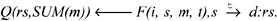Assume that the fact table granularity is "year," and the granularity of dim(s)is "day." A fact in an instance of F could be of the form <i1, s1, 100, 1998> The dimension may have a pair of tuples <s1, rs1, 1-1-1998, 5-5-1998> and <s1, rs2, 5-6-1998, 12-31-1998>. Thus, the fact would be counted twice.

Definition 10 (Mutual Recursion): Given a set R of TOLAP rules, a precedence graph G is built as follows: for each aggregate, predicate, or fact atom P, there is a node named P in G. If P and Q are nodes in G, add an edge from P to Q if there is a rule Γ in R such that P and Q occur in the body and the head of Γ, respectively. Following Abiteboul, Hull, & Vianu (1995), we say that two aggregate, fact, or predicate atoms R and S are mutually recursive if R and S participate in the same cycle in G.

Definition 11 (TOLAP Programs): A finite set of TOLAP rules which does not contain mutually recursive atoms is called a TOLAP program.

#### Semantics

We will use point-based semantics (Toman, 1995) for the roll-up functions. This means, for instance, that in a dimension such as Store of our running example, a value for a roll-up, say storeId:s‘i1’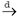city:c:‘c1,’ exists for each month in its validity interval.

Let us assume that for each dimension instance, we have a pair of relations, call them RD and DD, representing the sets TRUP and TDESC of Definition 2, respectively. The multidimensional database is defined over three different domains: D, N, TP, where variables ranging over D belong to an uninterpreted sort, the ones ranging over N belong to an interpreted sort (), and the temporal variables range over TP, as previously defined. For each dimension d, we will consider that TP is limited to the lifespan of d.

A valuation θ, for a TOLAP rule Γ, is a tuple (θS, θI), where θS is called a schema valuation, and θI is an instance valuation. Valuation θS maps the level and attribute variables in Γ to level and attribute names in BS(DS, FS), while θI maps domain variables to values in I(B).

Definition 12 (Valuations): A schema valuation for a rule Γ, denoted θS(Γ) maps level and attribute variables in the atoms of Γ as follows:

• given a roll-up atom of the form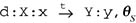maps d to a dimension name in Ds, t to a value w TP, and X and Y to a pair of values (v, u) s.t.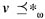u holds in d;

• if the roll-up atom is of the form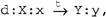, θS maps t to a value ω TP, and X and Y to a pair of values v, u s.t. v u holds in dim(X) Ds;

• if the roll-up atom is of the form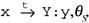, maps t to a value ω TP, and Yto a value u s.t. level(x,w)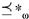holds in dim(X) Ds;

• for a roll-up atom of the form x Y:y, θS maps Yto a dimension level u in dim(Y), s.t.linf * u holds in dim(X);

• given a descriptive atom of the form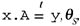maps t to a value ω Tp, and A to an attribute name u A, s.t. u>>t level (x,w) in dim(X) Ds.

Given a rule schema valuation θS(Γ) for a rule Γ, an instance valuation is a function θI s.t.:

• θI maps the domain variables x and y in the roll-up atoms defined above to values in RD over levels defined by θs;

• θI maps variable x in the descriptive atom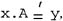, to values in DD, over levels defined by θs, and y to a value in D or N;

• θI maps a fact atom F(x1, , xn M, t) as follows: the rightmost term t in F is mapped to a value ω TP; each domain variable xi in F to a value in dom (level (xi, w)); and the data term M in F to a value in N ( M is the measure of the fact table);

• a constraint atom x {<, =} y evaluates to true whenever θI(x) {<, =} θI(y) is true. Recall that every variable in a constraint atom must appear in a roll-up or fact atom in the body. Thus, either θI maps x and y in the way described above, or they are mapped to a value in D, N or TP;

• a negatedroll-up atom is evaluated using the Close World Assumption. Thus, !(x Y:y)is true if, given a valuation θ s.t. θ(x) = u, θ(t) = ω, θ(Y) = l, and θ(y) = v, then there does not exist a roll-up in dim(x)s.t.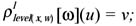recall that every variable must be in a positive atom in the body of the rule;

• a negated descriptive atom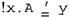is treated as explained above for negated roll-up atoms; thus,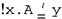is true if, given a valuation θ s.t. θ(x) = u, θ(t) =, ω, θ (A) = a, and θ(y) = v, a description does not exist in dim(x)such that ξ[t]level(x, w)a (u) = v;

• a negated constraint atom is evaluated in the standard way (i.e., θ(!x = b) =θ(x <> b));.

• a negated fact atom !F(x, y, , "a," m, t) is true if for a valuation θI(x) = u, θI(y) = v, , the tuple <u, v……. "a," > is not in F;

• predicate atoms are valuated as in standard data log (Abiteboul, Hull, & Vianu, 1995).

Let AGG be the set of aggregate functions, with extension AGG = {MIN, MAX, COUNT, SUM, AVG}, and r a relation. The aggregate operation (Consens & Mendelzon, 1990) γfA(X) (r) is the relation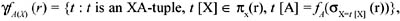over XA, s.t. XA schema (r), f AGG, and fA(r) denotes the aggregation of the values in t [A], t r, using t.

We can now define the semantics of a TOLAP rule Γ of the form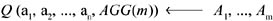as follows:
For each level or data variable vi in the body of Γ, and for a valuation θ of the variables in the rule's body, we have: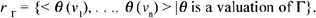Then Q = γAGGm(a1, , an) (rΓ)

#### Safety

Queries expressed as TOLAP rules studied in the previous subsections always lead to finite answers. This follows from the syntactic restrictions and from the semantics already defined. The existence of functions dim(x) and level (x,t) makes every variable in a rule bounded by the active domain of some level in a dimension. This is analogous to the concept of range restricted variable defined by Abiteboul, Hull, & Vianu (1995). Thus, for instance, if a negated roll-up atom appears in the body, safety is always granted. The same occurs with negated fact atoms. Also, limiting the temporal domain to the lifespan of the dimensions avoids infinite query answers. Thus, we conclude that TOLAP rules are safe.

#### What Can Be Expressed in TOLAP?

Intuitively, it is not hard to see that TOLAP has at least the power of first-order query languages with aggregation. However, in a sense it goes beyond this class. Note that in the temporal multidimensional data model, only the direct roll-ups are stored. Thus, to evaluate a roll-up atom like d:X:xY:y, we need to compute the transitive closure of the roll-up functions in dimension d. It is a well-known fact that this cannot be done in first order, even after adding aggregate functions (Libkin & Long, 1997). However, as long as the dimension schema is fixed, this computation can be done in first order, because for a fixed schema, the number of joins needed to transitively close the roll-up functions is known in advance.

Observe that not only the structure of a dimension is subject to updates. There are common real-life situations in which an instance of a dimension may be modified in a non-trivial fashion. For instance, suppose we add the Geography dimension of Figure 1 to our running example data warehouse, and pose the following query: "total sales per item and region, using only the currently existing regions." In TOLAP we would write: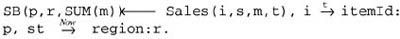The second roll-up atom filters out regions not valid today.

Suppose now the following constraint: if a region where a sale occurred does not exist today, we want in the result a descendant of such region, if it exists. This query cannot be expressed in TOLAP. To show this, suppose the Cuyo region is split into CuyoEast and CuyoWest. Later, CuyoEast is merged with another region, say Pampa. In the meantime, maybe some region could have been deleted. With the tools defined so far, we could not find the "descendants" of Cuyo, because this is a transitive closure problem even though the schema remains fixed. TOLAP can be extended in order to be able to express the class of queries exemplified above. This problem, however, is beyond the scope of this chapter.

### TOLAP Implementation

We now present a preliminary TOLAP implementation. We describe the data structure supporting the system, and how TOLAP atoms, rules, and programs are translated to SQL statements. We also discuss different implementation alternatives.

A first TOLAP implementation was developed at the University of Buenos Aires (Vaisman, 2001). Vaisman and Mendelzon (2001) give details of the implementation, and discuss preliminary results using a real-life case study, which we will briefly present in the next section.

#### Relational Representation

We will analyze two different data structures for representing temporal dimensions: a "fixed schema" versus a "non-fixed schema" approach. In both of them, a relation with schema (dimensionId, loLevel, toLevel, From, To) represents the structure of each dimension across time. Each tuple in this relation means that in the dimension identified by dimensionId, level loLevel rolled up to level toLevel between instants From and To. For example, the schema of the retail data warehouse will be represented by a relation with tuples like <D1, itemId, itemType, d1, Now>, <D1, itemId, brand, d2, Now>, and so on.

We briefly discuss how instances of the dimensions are stored under both kinds of representations.

Fixed Schema. In a fixed-schema relational representation, a dimension instance is a relation with tuples of the form (loLevel, upLevel, toVal, upVal, From, To). Each tuple represents a roll-up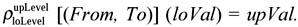As an example, the instances of dimension Product in our retail data warehouse would be represented by a relation with tuples of the form <itemId, itemType, i1, ty1, dl, d3>, <itemId, brand, i1, b1, d1, Now>, and so on. Thus, as new levels are added to a dimension, new tuples are inserted in the relation representing the dimension, but the relation's schema does not change. For instance, adding a new level company above brand just requires adding tuples like <brand, company, b1, c1, d6, Now> (of course, in the relation storing the schema, a tuple <D1, brand, company, d6, Now> must be inserted).

This is probably the most natural representation, because it implements the relation RD straightforwardly. However, translation to SQL would be awkward, and the resulting SQL queries will be far from optimal, because in order to compute aggregation over non-bottom dimension levels, the transitive closure of the roll-up functions must be computed, requiring self-joining temporal relations.

Non-fixed Schema. In this case there is also one relation for each dimension, but each dimension level is mapped to an attribute in this relation. A single tuple captures all the possible paths from an element in the bottom level, to the distinguished element "all." Two attributes, From and To, indicate, as usual in the temporal database field, the interval within which a tuple is valid. For example, a relation representing dimension Product will have schema: {itemId, itemType, brand, From, To}.

This structure requires more complicated algorithms for supporting dimension updates. For instance, adding a dimension level above a given one, or below the bottom level, induces a schema update of the relation representing the dimension instance: a new column will be added to this relation. If a level is deleted, no schema update occurs (i.e., the corresponding column is not dropped, in order to preserve the dimension's history. In the example above, a column company would be added. However, the translation process is simpler, and the subsequent query performance better, because computing the transitive closure of the roll-up functions reduces to a single relation scan.

Fixed vs. Non-fixed Schemas. To make the ideas above more clear, let us show how a TOLAP query is translated to SQL. Later, we will give full details of this process.

Consider the query "total sales by company," posed to the earlier example of a data warehouse. This query reads in TOLAP: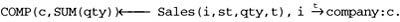Assuming that no schema update affected the fact table Sales, the SQL equivalent of the TOLAP query above in the fixed schema approach will look like :

`    SELECT P1.upLevel,SUM(sales)    FROM Sales S, Product P, Product P1, Time T    WHERE       S.itemId = P.loVal AND P.loLevel = 'itemId' AND       P.upLevel = 'brand' AND P1.upLevel = 'company' AND       P.upLevel = P1.loLevel AND       S.day between P.From AND P.To AND       S.day between P1.From AND P1.To    GROUP BY P1.upLevel `

In the non-fixed schema representation, the SQL equivalent for the query is:

`    SELECT P.company,SUM(sales)    FROM Sales S, Product P    WHERE       S.itemId = P.itemId AND       S.day between P.From AND P.To    GROUP BY P.company `

It is easy to see that the result is much more elegant and efficient in the second approach. The computation of the roll-up from itemId and company is straightforward, while in the first approach, the self-join of the table Product is required.

These arguments lead to us conclude that the non-fixed schema approach would be the better choice for a TOLAP implementation.

#### Translating TOLAP into SQL

The non-fixed schema approach discussed above was followed in the implementation of TOLAP. A parser performs a first pass over a rule, checking that the syntactic conditions of Definition 9 are met. In case an error is found, execution halts. In this first pass, the different atoms are identified and a symbol table built. The second pass translates each atom into an SQL statement and builds the equivalent SQL query.

We will show how a TOLAP rule of the form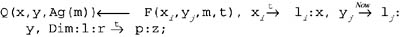is translated to SQL. The translation of atoms, rules, and programs will be explained in that order.

TOLAP Atoms. For each roll-up atom like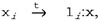, bound to a variable in a fact table, a selection clause is built as follows:

` F.i = Dimi.bottom AND F.time BETWEEN Dimi.From AND Dimi.To `

The expression Dimi is the table representing the dimension Di. The first conjunct joins this table with the fact table, on the attribute representing the bottom level of the dimension. The actual name of the column F.i is taken from the fact table's metadata. The second conjunct corresponds to the join between the fact table and the "Time" dimension.

• Each time constant is translated as a selection clause. The second roll-up atom in the rule above will be translated as:

` F.j = Dimj.bottom AND Dimj.To = Now `

Dimj is the dimension such that variable yj is bound to.

• If a roll-up atom of the form x Y:x corresponds to a user-defined time dimension, the atom is translated as:

` F.j = Dimj.bottom `
• A roll-up atom Dim:1: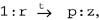, not bound to any fact table, is translated as an EXISTS clause.

` EXISTS    SELECT *    FROM Dim WHERE F.time between Dim.From AND Dim.To `

The WHERE clause is omitted if the join with the time dimension is not required.

• The roll-up from the bottom levels of the dimensions to the levels 1i and 1j, corresponding to the variables in the head of the rule above (the aggregation levels), is computed as a projection and an aggregation in the way shown below. Thus, the SQL query generated by the TOLAP query of the beginning of this section, will look like:

` SELECT Dimi.li, Dimj.lj,Ag(measure) FROM F_l, Dimi, Dimj WHERE    F_l.i = Dimi.bottom AND    F_l.j = Dimj.bottom AND    F_l.time between Dimi. From AND Dimj.To AND    Dimj.To = Now AND    EXISTS        (SELECT *         FROM Dim         WHERE         F_l.Time between Dim.From AND Dim.To) GROUP BY Dimi.li, Dimj.lj `

The term measure is the measure in the fact table, bound to variable m. The fact table sub-index represents the version of the fact table. In this case there is only one version, as no schema update occurred. We will come back to this shortly.

• A constraint atom is translated as a selection condition in the WHERE clause. If the constraint atom is negated, this condition is treated in the usual way (a NOT predicate is added).

• A negated roll-up atom is translated as a NOT EXISTS clause. Suppose that in the query above we add a negated atom as follows: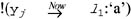where ‘a’ represents a constant. This atom is converted into an SQL expression of the form:

` NOT EXISTS(    SELECT *    FROM Dimj    WHERE    Dimj.To=Now AND Dimj.ll ='a' AND F.j = Dimj.bottom)    where ll is the attribute representing level ll. `
• A predicate atom is translated as a table in the FROM clause, with the conditions which arise from the variables or constants in it.

TOLAP Rules. So far we tackled the problem of translating each atom in a TOLAP rule separately. The next step will be the study of the translation of the whole rule.

Above, we gave an example of a simple rule, assuming no schema update occurred in the fact table. However, we claimed that one of the main features of TOLAP is the ability to deal with different schema versions triggered by the insertion or deletion of a bottom level. Given a fact table F, versions F1, F2, and so on may be created, each one with a different schema.

Given a TOLAP query involving a fact table F, with versions Fl ,, Fn, the SQL query Q equivalent to Γ will be such that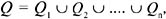where Qi are queries involving facts that occurred in the intervals Ii in which each F_i holds. If the query involves an aggregation function, call it fAGG, one more aggregation must be performed, in order to consider duplicates in each subquery. Thus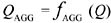TOLAP programs are treated analogously, as a series of TOLAP rules.

Join elimination. There are cases in which the join between dimensions and fact tables is not needed. This situation arises when a variable in the head of the rule belongs to a level which is the bottom level of a fact table in the body (or was the bottom level at least during some interval Ii). The current TOLAP implementation takes advantage of this fact, and does not generate the join.

Example 8:Let us consider the situation stated earlier in which the fact table Sales of our running example is split into Sales1, and Sales2, each one holding before and after an instant d5, as a result of the insertion of level storeId below level city. In TOLAP, the query "total sales by itemId and city" reads: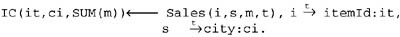Two SQL subqueries will be generated, one for each fact table.

` SELECT itemId,city,SUM(sales) FROM (   SELECT itemId,city, SUM(sales)   FROM Sales1   GROUP BY itemId,city   UNION ALL   SELECT itemId,city, SUM(sales)   FROM Sales2, Store   WHERE   Sales2.Time between Store.From AND Store.To AND   Sales2.storeId=Store.storeId GROUP BY itemId,city) GROUP BY itemId,city `

Notice that, as city and itemId were the bottom levels of Sales1, no join is needed in the first subquery.

Subquery Pruning. If a TOLAP rule contains a constraint atom with a condition over time such that the lifespan of a version of the fact table does not intersect with the interval determined by the constraint, the subquery corresponding to that version of the fact table is not generated by the translator, as it will not yield any tuple in the output. For instance, in Example 8, adding the constraint t < d6 will prevent the first subquery from being generated. We call this step subquery pruning.

### A Case Study

In order to illustrate the need for temporal management in OLAP discussed in this chapter, let us present a real-life case study, a medical center in Argentina (Vaisman & Mendelzon, 2001), where each patient receives different services, including radiographies, electrocardiograms, medicine, disposable material, and so on. These services are denoted "Procedures." Data was taken from different tables in the clinic's operational database. Figure 8 shows the final state of the dimensions. We built a temporal data warehouse using six months of data taken from medical procedures performed on patients at the center.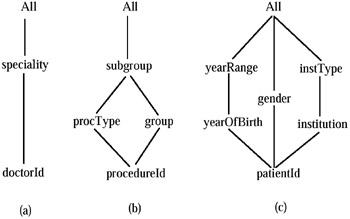Figure 8: Dimensions in the Case Study

Dimension Procedure, with bottom level procedureld, and levels procedureType, subgroup, and group, describes the different procedures available to patients. Dimension Patient, with bottom level patientId, and levels yearOfBirth and gender, represents information about the person under treatment. Age intervals are represented by a dimension level called yearRange. Patients are also grouped according to their health insurance institution. Moreover, these institutions are further grouped into types (level institutionType), such as private institutions, labor unions, etc. Dimension Doctor gives information about the available doctors (identified by doctorId) and their specialties (a level above doctorId). There is also a user-defined dimension Time, with levels day, week, and month.

A fact table holds data about procedures delivered to patients by a certain doctor on a certain date. We call this fact table Services, and its schema contains the bottom levels of the dimensions above, plus the measure. The fact table schema is: Services (doctorlD, procedureld, patientlD, day, quantity, t), where t represents a system-maintained time dimension, and quantity is the measure; attribute day represents a user-defined time dimension. For instance, a tuple <docl, 10.01.01, pat120, 10, 2, "10/10/2000"> means that doctor "doc1" prescribed two special radiographies to patient "pat120" on day "10" which corresponds to October 10, 2001. In this case study, the system-maintained and user-defined times are synchronized, meaning that there is a correspondence between the values of attributes "day" and "t" (e.g., day "11" corresponds to October 11, 2000). This synchronization, however, is not mandatory in the model. Thus, both times can take independent values.

The scenario was prepared as follows. Dimension Procedure was created with bottom level procedureId. Subsequent operations generalized this level to level subgroup. Level procedureId was then generalized to procType. Finally, subgroup was generalized to group, and procType related to group. In the Patient dimension, the initial bottom level patientId represents information about the person under treatment. This level was later generalized to year0fBirth, gender, and institution, in that order. Levels yearOfBirth and institution were further generalized into yearRange and institutionType, respectively. For dimension Doctor, in order to show how schema versioning is handled in TOLAP, we assumed that although facts were recorded at the doctorId level, this level was temporarily deleted, originating the second version of the fact table, called Services_2. Thus, during a short interval, the dimension's bottom level was specialty, later specialized into level doctorId, which triggered the creation of the third version of the fact table, denoted Services_3.

Finally, a user-defined Time dimension was created, with granularity day, allowing expressing aggregates over time. The dimension's hierarchy is the following: day rolls-up to week and month; week and month roll-up to All. The three resulting fact table versions have the following schemas: (a) Services_1:{procId, patientId, doctorId, day, quantity, tmp}, where tmp represents the built-in time dimension, and quantity is the number of procedures delivered; (b) Services_2:{procId, patientId, specialty, day, quantity, tmp}; (c) Services_3:{procId, patientId, doctorId, day, quantity, tmp}. The fact tables were populated off-line.

The following queries reflect TOLAP's expressive power. Suppose a user wants to analyze the doctors' workloads, in order to estimate future needs. The following query measures how the arrival of a new doctor influences the number of patients served by a doctor named Martinez: "list the total number of services delivered weekly by Dr. Martinez while Dr. Feinsilver was not working for the clinic."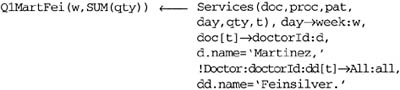Notice that the negated atom is not bound to the fact table. Also notice the use of the user-defined Time dimension.

The following query returns the number of services delivered by Dr. Martinez while both doctors were employed at the clinic.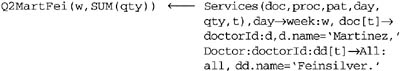The next query illustrates how to check patients who were served when they were affiliated to ‘MEDICUS’ and are currently affiliated to ‘OSDE’ (two private health services in Argentina).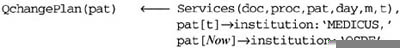The reader must notice that the queries above would require several lines of SQL code. Moreover, without an underlying temporal multidimensional data model, these queries cannot be expressed, unless an ad-hoc design of the data warehouse is carried out.

### Visualization

The TOLAP implementation includes a graphic environment developed taking advantage of the temporal multidimensional model. The graphic interface allows to:

• browse dimensions across time, and watch how they were hierarchically organized throughout their lifespan; further, dimension instances could also be browsed;

• import roll-up functions from text files;

• browse different versions of a fact table;

• send TOLAP programs to the system, and display their results without leaving the environment, including seeing the generated SQL query.

Figure 9 shows a typical system screen depicting a dimension Patient, taken from the case study presented above, as of December 13, 2000. The window on the left presents the dimension's structure. The arrows upon the window allow browsing forward and backward across time. This is not allowed if the system is in "initial" mode. The window on the right shows the dimension's instances. This window is synchronic with respect to the one on the left. Thus, the instances being displayed correspond to the structure on the left although they can vary as instance updates occur. However, while the screen of the left remains unchanged, several instance updates may occur, which can be displayed in the window on the right. The little box in the upper middle indicates the number of elements in the bottom level which are going to be displayed, allowing partial loading of the instance graph in main memory. This feature is crucial in making the tool usable in real applications, because loading the entire instance graph would be very expensive, even for not very large dimensions.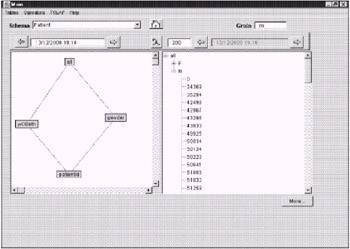Figure 9: Browsing Dimension "Patient"

For the sake of clarity we do not show here how time granularity is handled in the translation; usually, this will depend on the underlying DBMS.

Notation: The dimensions' sub-indices represent their position in the fact table to which they are bound. In the implementation, constructions of the form x 4 Y:x, are replaced by x[t] -+ Y:x, in order to simplify parsing.

In an actual implementation, Now can be replaced by Sysdate() or any function returning the current time.

Actually, the parenthesis is not required.Multidimensional Databases: Problems and Solutions
ISBN: 1591400538
EAN: 2147483647
Year: 2003
Pages: 150